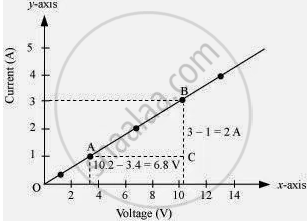Share

# Plot a Graph Between V and I and Calculate the Resistance of that Resistor. - CBSE Class 10 - Science

ConceptElectric Potential Difference

#### Question

The values of current I flowing in a given resistor for the corresponding values of
potential difference V across the resistor are given below –

 I (amperes) 0.5 1 2 3 4 V (volts) 1.6 3.4 6.7 10.2 13.2

Plot a graph between V and I and calculate the resistance of that resistor.

#### Solution

The plot between voltage and current is called IV characteristic. The voltage is plotted on x-axis and current is plotted on y-axis. The values of the current for different values of the voltage are shown in the given table.

 V (volts) 1.6 3.4 6.7 10.2 13.2 I (amperes ) 0.5 1 2 3 4

The IV characteristic of the given resistor is plotted in the following figure.The slope of the line gives the value of resistance (R) as,

Slope = 1/= BC/AC = 2/6.8

R= 6.8/2 = 3.4 Ω

Therefore, the resistance of the resistor is 3.4 Ω.

Is there an error in this question or solution?

#### Video TutorialsVIEW ALL 

Solution Plot a Graph Between V and I and Calculate the Resistance of that Resistor. Concept: Electric Potential Difference.
S